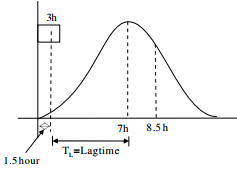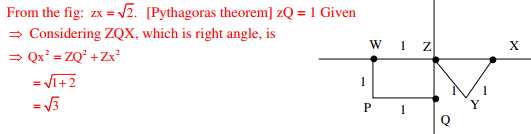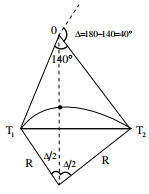# CE GATE 2014 PAPER 02 - Online Test

Q1. An isolated 3-h rainfall event on a small catchment produces a hydrograph peak and point of inflection on the falling limb of the hydrograph at 7 hours and 8.5 hours respectively, after the start of the rainfall. Assuming, no losses and no base flow contribution, the time of concentration (in hours) for this catchment is approximately
Explaination / Solution:For small catchment, time of concentration is equal to lag time of peak flow.
Tc =7−1.5=5.5h

Q2. The Muskingum model of routing a flood through a stream reach is expressed as O2 = K0I2 + K1I1 + K2O1, where K0, K1 and K2 are the routing coefficients for the concerned reach, I1 and I2 are the inflows to reach, and O1 and O2 are the are the outflows from the reach corresponding to time steps 1 and 2 respectively. The sum of K0, K1 and K2 of the model is
Explaination / Solution:
No Explaination.

Q3. The dominating microorganisms in an activated sludge process reactor are
Explaination / Solution:
No Explaination.

Q4. A road is being designed for a speed of 110 km/hr on a horizontal curve with a super elevation of 8%. If the coefficient of side friction is 0.10, the minimum radius of the curve (in m) required for safe vehicular movement is
Explaination / Solution:

= (110×110)/(127(0.08+0.10)) = 529.30m

Q5. X is 1 km northeast of Y. Y is 1 km southeast of Z. W is 1 km west of Z. P is 1 km south of W. Q is 1 km east of P. What is the distance between X and Q in km?
Explaination / Solution:Q6. The two air pollution control devices that are usually used to remove very fine particles from the flue gas are
Explaination / Solution:
No Explaination.

Q7. On a section of a highway the speed-density relationship is linear and is given by v = [80-(2/3)k]; where v is in km/h and k is in veh/km. The capacity (in veh/h) of this section of the highway would be
Explaination / Solution:Q8. The survey carried out to delineate natural features, such as hills, rivers, forests and manmade features, such as towns, villages, buildings, roads, transmission lines and canals is classified as
Explaination / Solution:

Topographic survey is carried out to delineate natural features.

Q9. As per Indian Standard Soil Classification System (IS: 1498 - 1970), an expression for A-line is
Explaination / Solution:
No Explaination.

Q10. The chainage of the intersection point of two straights is 1585.60 m and the angle of intersection is 140o . If the radius of a circular curve is 600.00 m, the tangent distance (in m) and length of the curve (in m), respectively are
Explaination / Solution:Length of curve, L = (π/180)× ∆×R
= (π/180)×40×600 = 418.88m
Tangent distance, T = R tan(∆/2)
= 600tan(40/2) = 218.38m